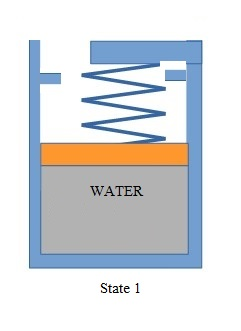# A piston-cylinder assembly is fitted with a linear spring (k = 40 kN/m) and a set of stops which...

## Question:

A piston-cylinder assembly is fitted with a linear spring (k = 40 kN/m) and a set of stops which limits the maximum internal volume of the cylinder to 50.5 L, the cross sectional area of the piston is 0.1 {eq}m^2 {/eq}. Initially, the cylinder contains 0.5 L of water at 100 kPa and 25{eq}^\circ {/eq}C (State 1). In this configuration, the linear spring is in contact with both the piston and the roof of the assembly (see figure).Heat is now added to the water, causing it to expand and raise the piston. The water reaches State 2 when the piston just hits the stops. From State 2, heat continues to be added until the pressure of the water reaches 3 MPa (State 3).

(a) What is the mass of the water (in kg)?

(b) What is the temperature of the water in State 2?

(c) What is the temperature of the water in State 3?

(d) Sketch the process (from State 1 to State 3) on a P-v diagram.

(e) Calculate the boundary work (in kJ) in going from State 1 to State 3.

## Hooke's Law:

In the applications of Hooke's spring, he directly correlates the spring force and the underlying change in length. Through experimentations and trials, he concluded that for the same materials, the force applied is directly proportional to the change in length. Hence from that, he computed the spring constant for materials.

Become a Study.com member to unlock this answer! Create your account

From Steam Tables:

At State 1( 100 kPa, 25 C)

{eq}h_1=105\enspace kJ/kg {/eq}

{eq}u_1=105\enspace kJ/kg {/eq}

{eq}v_1=0.001\enspace...Hooke's Law & the Spring Constant: Definition & Equation

from

Chapter 4 / Lesson 19
201K

After watching this video, you will be able to explain what Hooke's Law is and use the equation for Hooke's Law to solve problems. A short quiz will follow.